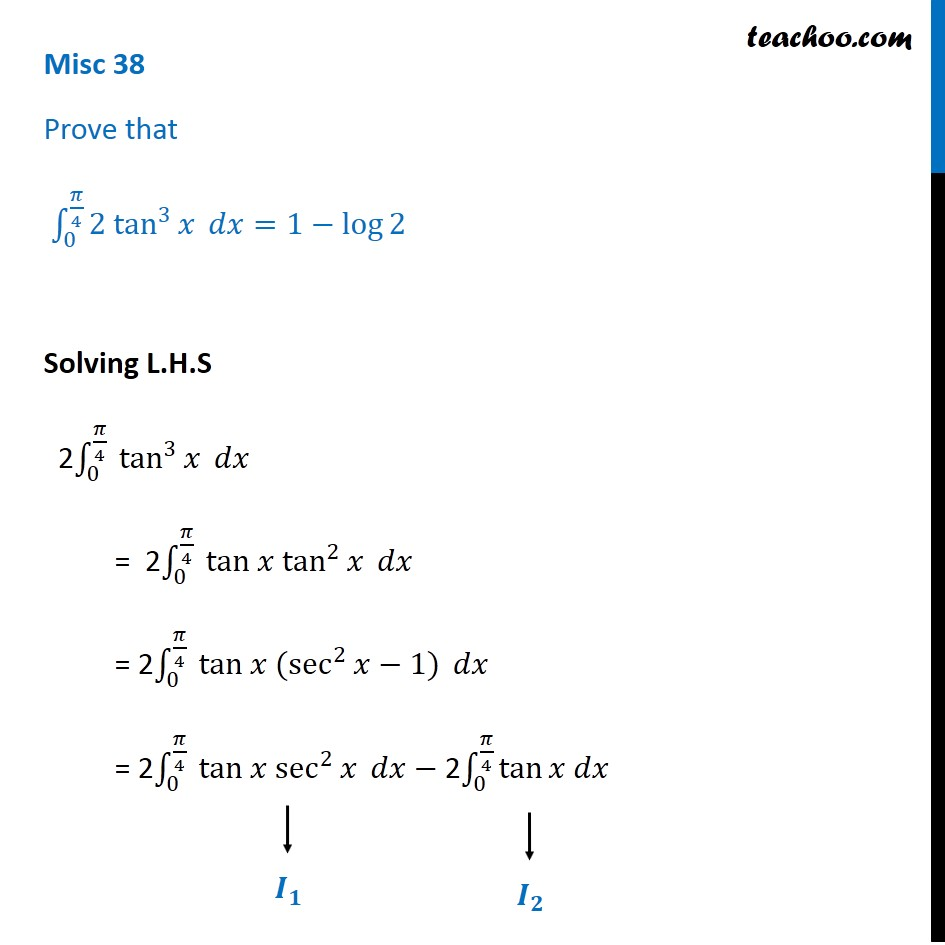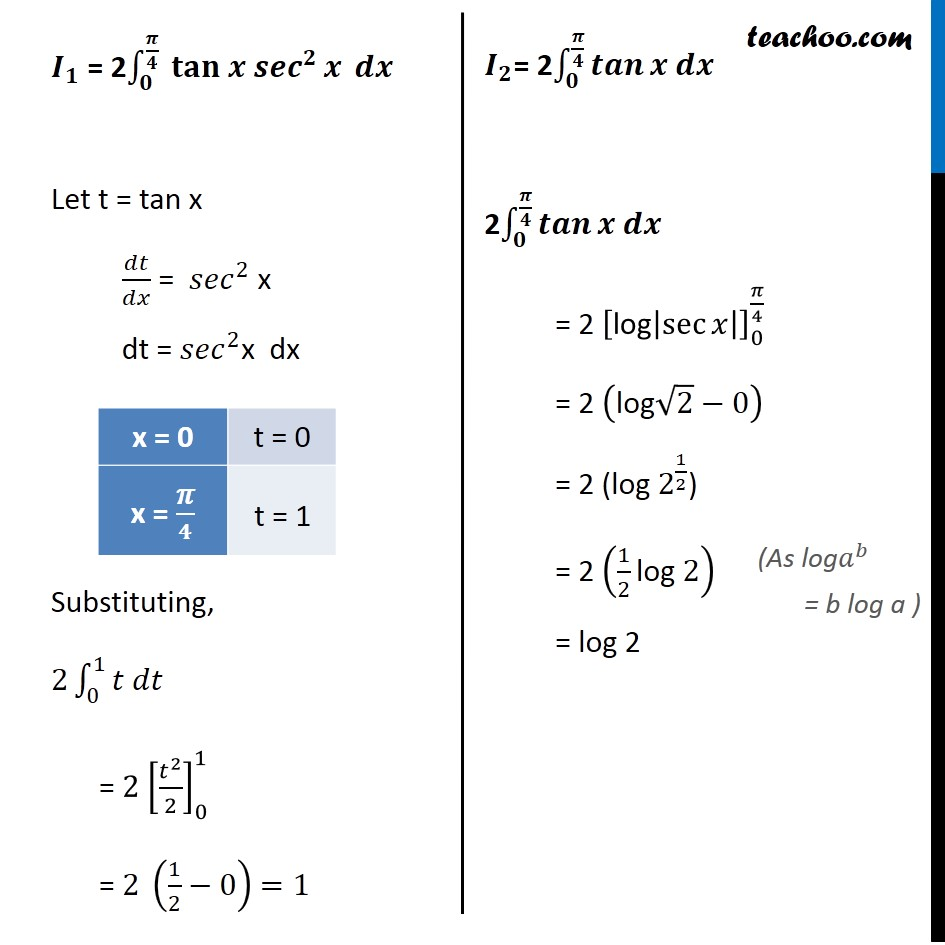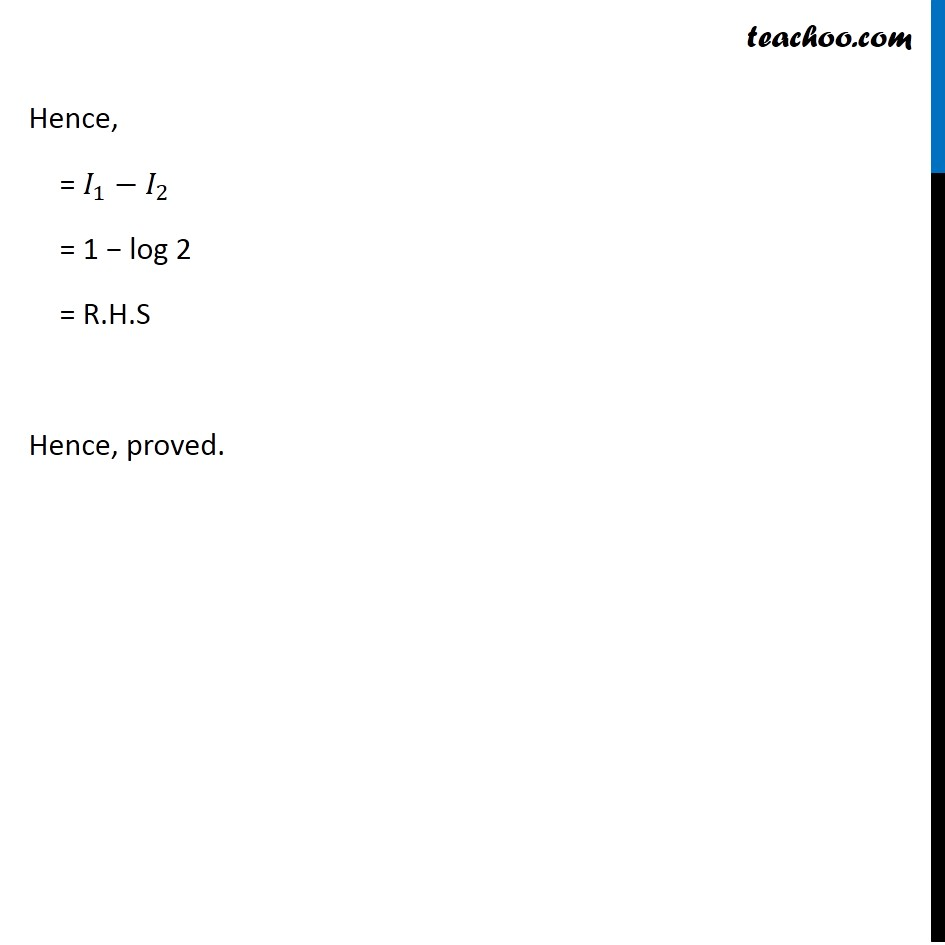Integration Full Chapter Explained - Integration Class 12 - Everything you need1. Chapter 7 Class 12 Integrals
2. Concept wise
3. Definite Integration - By Formulae

Transcript

Misc 38 Prove that ∫_0^(𝜋/4)▒〖2 tan〗^3⁡𝑥 𝑑𝑥=1−log⁡2 Solving L.H.S 2∫_0^(𝜋/4)▒〖 tan〗^3⁡𝑥 𝑑𝑥 = 2∫_0^(𝜋/4)▒〖 tan 𝑥 tan〗^2⁡𝑥 𝑑𝑥 = 2∫_0^(𝜋/4)▒〖 tan 𝑥 (sec〗^2⁡〖𝑥−1)〗 𝑑𝑥 = 2∫_0^(𝜋/4)▒〖 tan 𝑥 sec〗^2⁡𝑥 𝑑𝑥− 2∫_0^(𝜋/4)▒tan⁡〖𝑥 𝑑𝑥〗 𝑰_𝟏 = 2∫_𝟎^(𝝅/𝟒)▒〖 𝐭𝐚𝐧 𝒙 𝒔𝒆𝒄〗^𝟐⁡𝒙 𝒅𝒙 Let t = tan x 𝑑𝑡/𝑑𝑥 = 〖𝑠𝑒𝑐〗^2 x dt = 〖𝑠𝑒𝑐〗^2x dx Substituting, 2∫1_0^1▒〖𝑡 𝑑𝑡〗 = 2 [𝑡^2/2]_0^1 = 2 (1/2−0) =1 𝑰_𝟐= 2∫_𝟎^(𝝅/𝟒)▒𝒕𝒂𝒏⁡〖𝒙 𝒅𝒙〗 2∫_𝟎^(𝝅/𝟒)▒𝒕𝒂𝒏⁡〖𝒙 𝒅𝒙〗 = 2 ["log" |saec⁡𝑥 |]_0^(𝜋/4) = 2 ("log" √2−0) = 2 (log 2^(1/2)) = 2 (1/2 "log " 2) = log 2 Hence, = 𝐼_1−𝐼_2 = 1 − log 2 = R.H.S Hence, proved.

Definite Integration - By Formulae

About the AuthorDavneet Singh
Davneet Singh is a graduate from Indian Institute of Technology, Kanpur. He has been teaching from the past 9 years. He provides courses for Maths and Science at Teachoo.